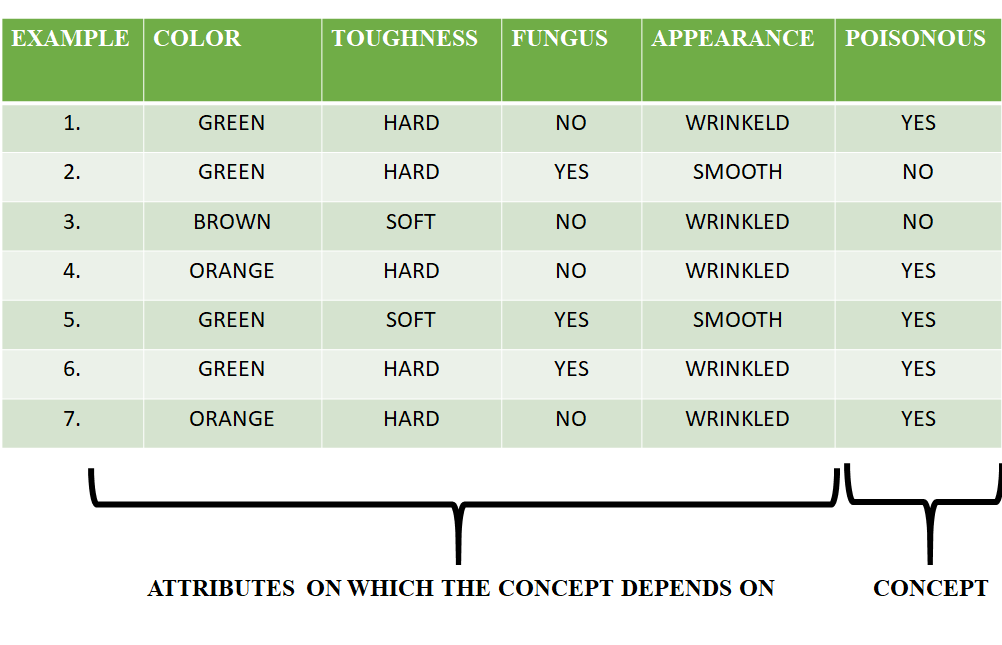Related Articles

# ML | Find S Algorithm

• Difficulty Level : Easy
• Last Updated : 10 Jun, 2021

Introduction :
The find-S algorithm is a basic concept learning algorithm in machine learning. The find-S algorithm finds the most specific hypothesis that fits all the positive examples. We have to note here that the algorithm considers only those positive training example. The find-S algorithm starts with the most specific hypothesis and generalizes this hypothesis each time it fails to classify an observed positive training data. Hence, the Find-S algorithm moves from the most specific hypothesis to the most general hypothesis.

Important Representation :

1. ? indicates that any value is acceptable for the attribute.
2. specify a single required value ( e.g., Cold ) for the attribute.
3. ϕindicates that no value is acceptable.
4. The most general hypothesis is represented by: {?, ?, ?, ?, ?, ?}
5. The most specific hypothesis is represented by: {ϕ, ϕ, ϕ, ϕ, ϕ, ϕ}

Steps Involved In Find-S :

h = {ϕ, ϕ, ϕ, ϕ, ϕ, ϕ}
2. Take the next example and if it is negative, then no changes occur to the hypothesis.
3. If the example is positive and we find that our initial hypothesis is too specific then we update our current hypothesis to a general condition.
4. Keep repeating the above steps till all the training examples are complete.
5. After we have completed all the training examples we will have the final hypothesis when can use to classify the new examples.

Example :

Consider the following data set having the data about which particular seeds are poisonous.First, we consider the hypothesis to be a more specific hypothesis. Hence, our hypothesis would be :
h = {ϕ, ϕ, ϕ, ϕ, ϕ, ϕ}

Consider example 1 :

The data in example 1 is { GREEN, HARD, NO, WRINKLED }. We see that our initial hypothesis is more specific and we have to generalize it for this example. Hence, the hypothesis becomes :
h = { GREEN, HARD, NO, WRINKLED }

Consider example 2 :
Here we see that this example has a negative outcome. Hence we neglect this example and our hypothesis remains the same.
h = { GREEN, HARD, NO, WRINKLED }

Consider example 3 :
Here we see that this example has a negative outcome. Hence we neglect this example and our hypothesis remains the same.
h = { GREEN, HARD, NO, WRINKLED }

Consider example 4 :
The data present in example 4 is { ORANGE, HARD, NO, WRINKLED }. We compare every single attribute with the initial data and if any mismatch is found we replace that particular attribute with a general case ( ” ? ” ). After doing the process the hypothesis becomes :
h = { ?, HARD, NO, WRINKLED }

Consider example 5 :
The data present in example 5 is { GREEN, SOFT, YES, SMOOTH }. We compare every single attribute with the initial data and if any mismatch is found we replace that particular attribute with a general case ( ” ? ” ). After doing the process the hypothesis becomes :
h = { ?, ?, ?, ? }
Since we have reached a point where all the attributes in our hypothesis have the general condition, example 6 and example 7 would result in the same hypothesizes with all general attributes.
h = { ?, ?, ?, ? }

Hence, for the given data the final hypothesis would be :
Final Hyposthesis: h = { ?, ?, ?, ? }

Algorithm :

```1. Initialize h to the most specific hypothesis in H
2. For each positive training instance x
For each attribute constraint a, in h
If the constraint a, is satisfied by x
Then do nothing
Else replace a, in h by the next more general constraint that is satisfied by x
3. Output hypothesis h ```

Attention reader! Don’t stop learning now. Get hold of all the important Machine Learning Concepts with the Machine Learning Foundation Course at a student-friendly price and become industry ready.

My Personal Notes arrow_drop_up